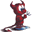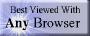music# subtraction

## summary

This subchapter looks at subtraction.

## free computer programming text book project

If you like the idea of this project,### stub section

This subchapter is a stub section. It will be filled in with instructional material later. For now it serves the purpose of a place holder for the order of instruction.

Professors are invited to give feedback on both the proposed contents and the propsed order of this text book. Send commentary to Milo, PO Box 1361, Tustin, California, 92781, USA.

# subtraction

This subchapter looks at subtraction.

### number systems

Here is a little bit of college level work on subtraction.

A system of numbers os considered to be closed under an operation if it reproduces itself.

S is a set of numbers. S is closed with respect to subtraction if for any two numbers a and b in the set S, the difference a - b is also a number in the set S. A set closed with respect to subtraction is called a modul.

The set of all integers {0, ±1, ±2, ±3, …} is a modul. The set of natural numbers {1, 2, 3, …} is not a modul.

The set of all even integers {0, ±2, ±4, ±6, …} is a modul.

The set of all rational numbers is a modul.

The set of all real numbers is a modul.

The set of all complex numbers is a modul.

The set of all purely imagiunary numbers ib is a modul.

A theorem regarding moduls:

(a) A modul always contains the number zero (0).

(b) If a modul contains the number a then it also contains the number -a.

(c) A modul is always closed with respect to addition.

Another theorem regarding moduls:

Any modul M containing only integers includes all multiples of the greatest common divisor of the numbers in M.

### assembly language instructions

For most processors, integer arithmetic is faster than floating point arithmetic. This can be reversed in special cases such digital signal processors.

On many processors, floating point arithmetic is in an optional unit or optional coprocessor rather than being included on the main processor. This allows the manufacturer to charge less for the business machines that don’t need floating point arithmetic.

The basic four integer arithmetic operations are addition, subtraction, multiplication, and division. Arithmetic operations can be signed or unsigned (unsigned is useful for effective address computations). Some older processors don’t include hardware multiplication and division. Some processors don’t include actual multiplication or division hardware, instead looking up the answer in a massive table of results embedded in the processor.

A specialized, but common, form of subtraction is an decrement instruction, which subtracts one from the contents of a register or memory location. For address computations, “decrement” may mean the subtraction of a constant other than one. Some processors have “short” or “quick” subtraction instructions that extend decrement to include a small range of values.

• SUB Subtract; DEC VAX; signed subtraction of scalar quantities (8, 16, or 32 bit integer or 32, 64, or 128 bit floating point) in general purpose registers or memory, available in two operand (first operand subtracted from second operand with result replacing second operand) and three operand (first operand subtracted from second operand with result placed in third operand) (SUBB2 integer subtract byte 2 operand, SUBB3 integer subtract byte 3 operand, SUBW2 integer subtract word 2 operand, SUBW3 integer subtract word 3 operand, SUBL2 integer subtract long 2 operand, SUBL3 integer subtract long 3 operand) (SUBF2 subtract float 2 operand, SUBF3 subtract float 3 operand, SUBD2 subtract double float 2 operand, SUBD3 subtract double float 3 operand, SUBG2 subtract G float 2 operand, SUBG3 subtract G float 3 operand, SUBH2 subtract H float 2 operand, SUBH3 subtract H float 3 operand); clears or sets flags
• SUB Subtract Integers; Intel 80x86; integer subtraction of the contents of a register or memory (8, 16, or 32 bits) from a memory location or a register; sets or clear flags
• SUB Subtract; Motorola 680x0, Motorola 68300; signed subtract of the contents of a data register (8, 16, or 32 bits) from a memory location or subtracts the contents of a memory location (8, 16, or 32 bits) from a data register; sets or clear flags
• SUB Subtract; MIX; subtract word or partial word field contents of memory from A-register (accumulator), overflow toggle possibly set
• SR Subtract Register; IBM 360/370; RR format; signed subtract of the contents of a general purpose register (32 bits) from a general purpose register (32 bits); register to register only; sets or clears flags
• S Subtract; IBM 360/370; RX format; signed subtract of the contents of a memory location (32 bits) from a general purpose register (32 bits); main memory to register only; sets or clears flags
• SH Subtract Half-word; IBM 360/370; RX format; signed subtract of the contents of a memory location (16 bits) from a general purpose register (low order 16 bits); main memory to register only; sets or clears flags
• SUBA Subtract Address; Motorola 680x0, Motorola 68300; unsigned subtract of the contents of a memory location or register (16 or 32 bits) from an address register; does not modify flags
• SUBI Subtract Immediate; Motorola 680x0, Motorola 68300; signed subtract of immediate data (8, 16, or 32 bits) from a register or memory location; sets or clears flags
• SUBQ Subtract Quick; Motorola 680x0, Motorola 68300; signed subtract of an immediate value of 1 to 8 inclusive from a register or memory lcoation; sets or clears flags for data registers and memory locations, does not modify flags for an address register
• DEC Decrement; DEC VAX; decrements the integer contents of a general purpose register or contents of memory (DECB byte, DECW word, DECL longword); equivalent to SUBx2 #1, sum, but shorter and executes faster; clears or sets flags
• DEC Decrement by 1; Intel 80x86; decrements the contents of a register or memory (8, 16, or 32 bits); sets or clear flags (does not modify carry flag)
• SBWC Subtract With Carry; DEC VAX; integer subtraction (32 bit) in general purpose registers or memory, first operand and the C (carry) flag subtracted from second operand with result replacing second operand; used for extended precision subtraction; clears or sets flags
• SBB Subtract Integers with Borrow; Intel 80x86; integer subtraction of the contents of a register or memory (8, 16, or 32 bits) and carry flag from a memory location or a register; sets or clear flags
• SUBX Subtract Extended; Motorola 680x0, Motorola 68300; (signed subtract of a data register [8, 16, or 32 bits] and the extend bit from a data register) or (signed subtract of the contents of memory location [8, 16, or 32 bits] and the extend bit from the contents of another memory location while predecrementing both the source and destination address pointer registers), used to implement multi-precision integer arithmetic; sets or clears flags

# free music player coding example

Coding example: I am making heavily documented and explained open source code for a method to play music for free — almost any song, no subscription fees, no download costs, no advertisements, all completely legal. This is done by building a front-end to YouTube (which checks the copyright permissions for you).

View music player in action: www.musicinpublic.com/.

Create your own copy from the original source code/ (presented for learning programming).

## view text bookHTML file

Because I no longer have the computer and software to make PDFs, the book is available as an HTML file, which you can convert into a PDF.

 Tweets by @osdata

## free computer programming text book project

Building a free downloadable text book on computer programming for university, college, community college, and high school classes in computer programming.

If you like the idea of this project,

send donations to:
Milo
PO Box 1361
Tustin, California 92781

Supporting the entire project:

If you have a business or organization that can support the entire cost of this project, please contact Pr Ntr Kmt (my church)

Some or all of the material on this web page appears in theThis web site handcrafted on Macintoshcomputers using Tom Bender’s Tex-Edit Plusand served using FreeBSD.†UNIX used as a generic term unless specifically used as a trademark (such as in the phrase “UNIX certified”). UNIX is a registered trademark in the United States and other countries, licensed exclusively through X/Open Company Ltd.

Names and logos of various OSs are trademarks of their respective owners.